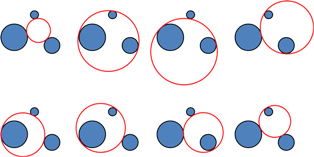# Apollonius ProblemAllPages RecentChanges Links to this page Edit this page Search Entry portal Advice For New Users

Apollonius' Problem is to draw a circle which touches (is a tangent to) another 3 objects - either points (P), lines (L) or circles (C).

The most general case is the circle which is a tangent to 3 other circles (CCC) when there are 8 solutions.The construction of these circles is not a trivial task.

The method is given at: http://mathworld.wolfram.com/ApolloniusProblem.html and clarification can be found at:

www.ajur.uni.edu/v3n1/Gisch and Ribando.pdf

The case of the circle touching 3 points (PPP) is the classical circumcircle of a triangle and is a unique solution.

There are 8 other cases PPL, CPL etc. which have differing numbers of solutions and complexity of construction.

Construction of the solution to these problems is described at: http://en.wikipedia.org/wiki/Special_cases_of_Apollonius'_problem

Most of the techniques required are hotlinked in these page however

describes carefully the necessary technique for forming the four tangents to two circles.

The free online drawing package Geogebra makes the investigation of these problems an easier exercise.